# hypocycloid

## roulette

last updated: 2005-03-25The curve is formed by the locus of a point, attached to a circle, that rolls on the inside 1) of another circle 2). In the curve's equation the first part denotes the relative position between the two circles, the second part denotes the rotation of the rolling circle.

The value of the constant b determines the starting point in relation to the circle:

The curve has a closed form when the ratio of the radius of the rolling circle and the radius of the other circle (a) is equal to a rational number. When giving this ratio its simplest form, the numerator is the number of revolutions inside the resting circle, before the curve closes. The denominator is the number of rotations of the rolling circle before this happens.
In this rational case the curve is algebraic, otherwise transcendental.

We let the rolling circle be smaller than the rolled circle 3), so that a < 1. And because the curve for a* = 1 - a is the same as for a, we can state that 0 < a <= 1/2.

A hypocycloid with parameters a and b is the same as a epicycloid with parameters a-1 and 1/b.

## (ordinary) hypocycloid

The resulting curve is a kind of star with a number of cusps. This amount is equal to the numerator of the constant a.

Some special hypocycloids are the following:

• a = 1/4: astroid
• a = 1/3: deltoid
• a = 1/2: line
This result has been found by the Persian astronomer and mathematician Nasir Al-Din al-Tusi (1201-1274). Therefore this solution has also the name of a Tusi couple.
• a = 1: circle
• a = 3/2: nephroid
• a = 2: cardioid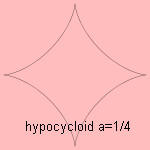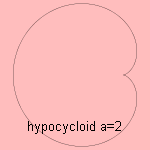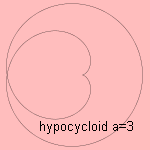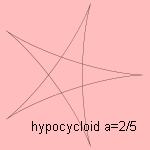Related curves:

The curve is a cycloidal curve.

## hypotrochoid4)

Now the point being followed is not lying on the rolling circle. When the point lays outside the circle (b>1), the curve is called a prolate hypocycloid. When the point lays inside the rolling circle (<1), the curve is called a curtate hypocycloid.

When the rolling circle is large than the rolled circle, the latter is on the inside of the rolling circle. In this situation the curve has also the name of a peritrochoid.

With a spirograph set many hypotrochoids can be drawn.

For a=1/3, b=1/2, we see an easy way to drill a hole with three edges.

When the factors for the two terms in the parameter equation for x are equal, the resulting curve is a rhodonea.
In this case holds b = 1/a - 1.

The Wankel rotary engine uses the the hypotrochoid principle. The rotary engine has a triangular rotor surrounded by a peripheral housing. This combination completes the same four cycles of intake, compression, combustion and exhaust and replaces the pistons, crankshafts cams and valves of the four stroke engine.
The inside of the triangular rotor has the form of a circle, that rolls on the inside a the second (smaller) circle. The rolling circle is 3/2 as large as the fixed one (so a=3/2).
Then, the three points of the triangular rotor make a hypotrochoid path (take e.g. b=13/6).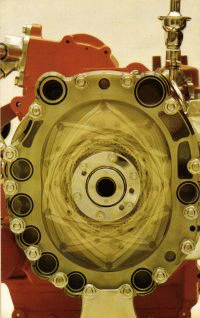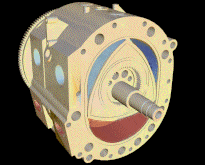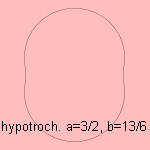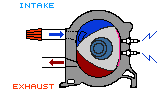Some examples: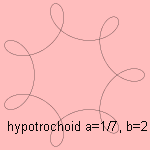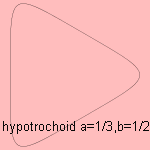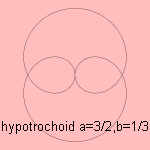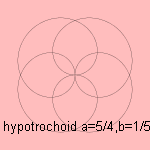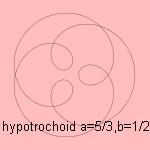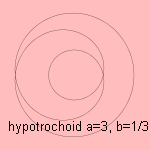notes

1) Hypo (Gr.) = under

2) Let a circle with radius r roll on the inside of a circle with radius R. Take as origin the center of the rolled circle. Now let the starting point be on a distance b r from the center of the rolling circle. Then the coordinates of the hypocycloid as a function of the rolled angle t are:3) If not, the rolling circle and the rolled circle can be interchanged.

4) Trochus (Lat.) = hoop.
Sometimes the meaning of hypocycloid and hypotrochoid is interchanged: hypotrochoid for the general case, hypocycloid only for the situation that the starting point is lying on the circle.